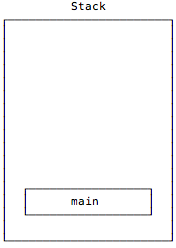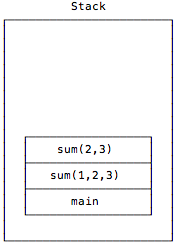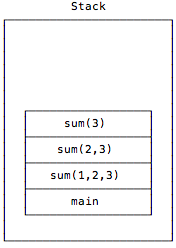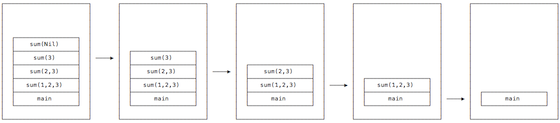# A Visual Look at JVM Stacks and Frames

Given the background information of the previous lesson, let’s take a visual look at how the JVM stack and stack frames work by going back to our recursive sum function from the previous lesson.

Before the `sum` function is initially called, the only thing on the call stack is the application’s `main` method:Then main calls `sum` with `List(1,2,3)`, which I show here without the “List” to keep things simple:The data that’s given to `sum` matches its second `case` expression, and in my pseudocode, that expression evaluates to this:

``return 1 + sum(2,3)``

Next, when a new instance of `sum` is called with `List(2,3)`, the stack looks like this:Again the second `case` expression is matched inside of `sum`, and it evaluates to this:

``return 2 + sum(3)``

When a new instance of `sum` is called with the input parameter `List(3)`, the stack looks like this:Again the second `case` expression is matched, and that code evaluates to this:

``return 3 + sum(Nil)``

Finally, another instance of `sum` is called with the input parameter `List()` — also known as `Nil` — and the stack now looks like this:This time, when `sum(Nil)` is called, the first `case` expression is matched:

``case Nil => 0``

That pattern match causes this `sum` instance to return `0`, and when it does, the call stack unwinds and the stack frames are popped off of the stack, as shown in this series of images:In this process, as each sum call returns, its frame is popped off of the stack, and when the recursion completely ends, the main method is the only frame left on the call stack. (The value `6` is also returned by the first `sum` invocation to the place where it was called in the `main` method.)

I hope that gives you a good idea of how recursive function calls are pushed-on and popped-off the JVM call stack.

## Manually dumping the stack with the sum example

If you want to explore this in code, you can also see the series of `sum` stack calls by modifying the `sum` function. To do this, add a couple of lines of code to the `Nil` case to print out stack trace information when that `case` is reached:

``````def sum(list: List[Int]): Int = list match {
case Nil => {
// this manually creates a stack trace
stackTraceAsArray.foreach(println)
// return 0 as before
0
}
case x :: xs => x + sum(xs)
}``````

Now, if you call `sum` with a list that goes from 1 to 5:

``````val list = List.range(1, 5)
sum(list)``````

you’ll get this output when the `Nil` case is reached:

``````java.lang.Thread.getStackTrace(Thread.java:1588)
recursion.SumWithStackDump\$.sum(SumWithStackDump.scala:19)
recursion.SumWithStackDump\$.sum(SumWithStackDump.scala:19)
recursion.SumWithStackDump\$.sum(SumWithStackDump.scala:19)
recursion.SumWithStackDump\$.sum(SumWithStackDump.scala:19)
recursion.SumWithStackDump\$.sum(SumWithStackDump.scala:19)``````

While that output isn’t too exciting, it shows that when the stack dump is manually triggered when the `Nil` case is reached, the `sum` function is on the stack five times. You can verify that this is correct by repeating the test with a `List` that has three elements, in which case you’ll see the `sum` function referenced only three times in the output:

``````java.lang.Thread.getStackTrace(Thread.java:1588)
recursion.SumWithStackDump\$.sum(SumWithStackDump.scala:13)
recursion.SumWithStackDump\$.sum(SumWithStackDump.scala:19)
recursion.SumWithStackDump\$.sum(SumWithStackDump.scala:19)``````

Clearly the `sum` function is being added to the stack over and over again, once for each call.

I encourage you to try this on your own to become comfortable with what’s happening.

## Summary: Our current problem with “basic recursion”

I hope this little dive into the JVM stack and stack frames helps to explain our current problem with “basic recursion.” As mentioned, if I try to pass a `List` with 10,000 elements into the current recursive `sum` function, it will generate a `StackOverflowError`. Because we’re trying to write bulletproof programs, this isn’t good.

## What’s next

Now that we looked at (a) basic recursion with the `sum` function, (b) how that works with stacks and stack frames in the last two lessons, and (c) how basic recursion can throw a `StackOverflowError` with large data sets, the next lesson shows how to fix these problems with something called “tail recursion.”